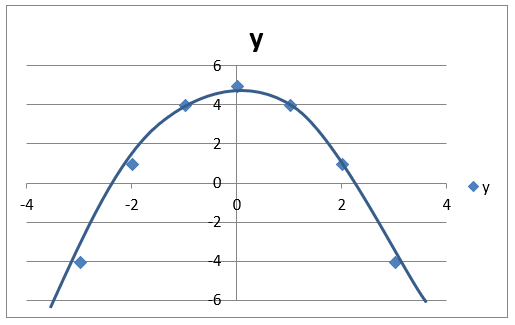## Pricing Table Particle

• Basic
• Standard Compliant Channels
• $50 • Completely synergize resource taxing relationships via premier market • 1 GB of space • Support at$25/hour
• Standard Compliant Channels
• $100 • Completely synergize resource taxing relationships via premier market • 10 GB of space • Support at$15/hour
• Platinum
• Standard Compliant Channels
• $250 • Completely synergize resource taxing relationships via premier market • 30 GB of space • Support at$5/hour

Calculus
Mathematics
Topic Fifteen

### Differentiation

Calculus is the study of change or changing quantities. Mathematical topics besides Calculus, like Arithmetic, Algebra, Geometry and Trigonometry involve unchanging quantities. In algebra, for example, any unknown quantities are usually fixed real numbers that we wish to solve for. Calculus is based on differentiation and integration. Therefore, the main use for differentiation is to find the gradient of a function at any point on the graph.

Differentiation is the process of finding a derivative, the calculus name for the slope or steepness of a curve. The slope is a simple rate, like kilometers per hour. Slope, as we learned in algebra, is the change in y divided by the change in x, or rise over run. As we will see, the slope for any shape (except a straight line) is constantly changing but can be described as an equation. In calculus, we look for the instantaneous rate of change - the slope of the line tangent to a curve at a single point.

Integration is used to find the area between the graph of a curve and the x-axis. It is really the process of cutting up an area into small sections and then adding up all of these small sections to get the total area. For example, you can easily calculate the area of a simple rectangle, but to calculate the area under a curve it is difficult because you do not have an area formula for this unusual shape. Differentiation can be used in this case.

Most of our discussion will be on differential calculus. In differential calculus, the basic problem is finding the slope of the tangent line to a curve at a given point on the curve. This problem is the core of differential calculus since this slope tells how fast the output variable of the function is changing with respect to the input variable. Solving this problem lets us find the instantaneous rate of change of y=f(x) with respect to x, which is the derivative. The slope of a line connecting two points is a ratio of the rise to the run, which is a ratio of the vertical distance between the points to the horizontal distance between the two points.

### The process of differentiation

Consider the following two functions:

"$y = x$"

"$y = x^2$"

The first function is a linear function and so the graph will produce a straight line.  The second function is not linear and the graph will not be a straight line but a u-shaped curve.

We can find the slope or coefficient of both of the above functions.  We can do this by differentiation.  In the first function, the slope of the line as mentioned above is constant and will not change for changes in output.  Therefore, for the function "$y = x$", the slope is 1 or constant.  If "$x$" increases by "$m$", "$y$" will change by exactly "$m$" so "$m = 1$".  However, in the function "$y = x^2$", if "$x$" changes by m, y will change by 2 not 1

This is so because the process of differentiation requires that the exponent is brought before the variable and 1 is minus from the original exponent.  For example,

If "$y = x^2$",

then the differentiation of this function is: "$2x$".  or "$2x^{2-1=1} = 2x^1= 2x$".  The numerical value before the "$x$" is the coefficient which is the slope.  The differentiation of "$y = x$"

is "$y = 1x^{1-1=0}$"

"$y = x^0$"

"$y = 1$".

### Basic Rules for Differentiation

1. The Power Rule

This is simply the example that we used above which is bringing the exponent before the variable and subtracting 1 from the original exponent.

 Examples: Function Derivative "$y = x^10$" "$y = -x^2$" "$y = \sqrt{x} \text{ or } \left(x^{\frac{1}{2}}\right)$" "$y=3x^2$" "$y = -7x^5$" "$y = \frac{1}{2}x^9$" "$y' = 10x^{10-1=9} = 10x^9$" "$y’ = -2x^{2-1=1} = -2x$" "$y’ = \frac{1}{2}x^{\frac{1}{2} -1 = -\frac{1}{2}} = \frac{1}{2} x^{-\frac{1}{2}}$" "$y’ = 2\left( 3 \right) x^{2-1=1} = 6x$" "$y’ = - 5 \left( 7 \right) x^{5-1=4} = -35x^4$" "$y’ = \frac{9}{2} x^8$"

 Examples: "$y = x^3 + 6x^4$" "$y = \frac{1}{6}x^2 + 12x^5$" "$y = 3x^4 + 10x^2 + 9x^4$" "$y’ = 3x^2 + 24x^3$" "$y’ = \frac{1}{3}x + 60x^4$" "$y’ = 12x^3 + 20x + 36x^3$" The derivative of a constant is zero and a constant is a number by itself. "$y = x^3 + 8$" "$y = \frac{1}{3}x^3 – 5$" "$y’ = 3x^2 + 0 = 3x^2$" "$y’ = x^2 - 0 = x^2$"
3. Product Rule

f times derivative of g + g times the derivative of f,

where the first part of the problem is f and the second part is g.

Example:

"$y = \left( 8x + 4 \right) \left( 2x^3 + 6 \right)$"

Solution:

In this case, "$\left( 8x + 4 \right)$" is “f “ and "$\left( 2x^3 + 6 \right)$" is the “g”.

So the derivative of the above is as follows where we use the normal power rule:

"$\left( 8x+4 \right) \left( 6x^2 \right) + \left( 2x^3 + 6 \right) \left( 8 \right) =$"

"$48x^3 +24x^2 + 16x^3 + 48 =$"

"$64x^3 + 24x^2 + 48$"

4. Quotient Rule

In this case, the rule is g times derivative of f - f times the derivative of g divided by g2 where g is the denominator and f is the numerator.

 Examples: "\begin{align} \\ y & = \frac{3 - 2x}{3 + 2x} \\ y’ & = \frac{ \left( 3+2x \right) - 2 - \left( 3 - 2x \right) • \left(2 \right)}{ \left( 3+2x \right)^2} \\ & = \frac{ \left( -6 \right) - \left( 4x \right) - 6 + 4x}{ \left( 3 + 2x \right)^2} \\ & = \frac{-12}{\left(3 + 2x \right)^2} \end{align}"
5. Chain Rule - function of a function – this is the power rule times the derivative of the function inside of the brackets.

Example:

"$y = \left( 2x + 8 \right) ^ 4$"

Solution:

"$y = 4 \left( 2x + 8 \right) ^ {3} • 2$"

Example: "$y = \sqrt{\left( 1 – 9x \right)}$"

Solution: "$y = \left( 1 – 9x \right) ^ {\frac{1}{3}}$"

"$y’ = \frac{1}{3} \left( 1 – 9x \right) ^ {\frac{-2}{3}}$" times "$-9$"

### Using Differential to find the Equation of a Line

Having found the gradient at a specific point using differentiation, we can use our coordinate geometry skills to find the equation of the tangent to the curve.

Procedure:

1. Differentiate the function.
2. Put in the x-value into dy/dx to find the gradient of the tangent.
3. Put in the x-value into the y function to find the coordinates of the point where the tangent touches the curve.
4. Put these values into the formula for a straight line:
"$y - y_1 = m \left( x - x_1 \right)$"
where "$m = \text{gradient}$" and "$\left( x_1, y_1 \right)$" is where the tangent meets the curve.

Example:

Find the equation of the tangent to the curve "$y = x^2 - 4x - 5$", when "$x = -1$":

Solution:

Finding the derivative of the above gives:

"$\frac{dy}{dx} = 2x – 4$" and so when "$x = -1$"

"$\frac{dy}{dx} = -6$" which is the slope or "$m$".

When "$x = -1$" and when we substitute this "$x$" into the original equation:

"$y = x^2 - 4x - 5$"

we get "$y = 1 + 4 - 5 = 0$" giving "$x$" and "$y$" values of "$\left( -1, 0\right)$"

Therefore, the equation of the tangent using the equation:

"$y - y_1 = m \left( x - x_1 \right)$" is

"$y − 0 = -6 \left( x - -1 \right)$" is

"$y − 0 = -6 \left( x + 1\right)$"

resulting in:

"$y = -6x - 6$"

So now we know that "$y = -6x - 6$" is the equation of the tangent at "$\left( -1, 0 \right)$".

### Stationary Points

Differentiation is useful to find one of the main features of the graph which is the stationary points.

These are points where the gradient = 0.

There are three types of stationary point: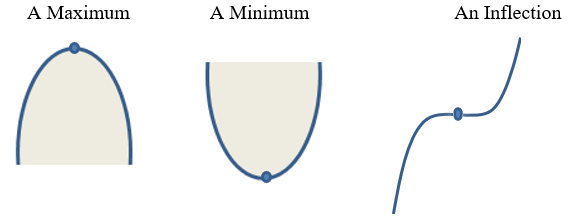Stationary points can be found by finding the second derivative which is denoted as:

"$\frac{d^{2}y}{dx^2}$"

The criteria are as follows:

If "$\frac{d^{2}y}{dx^2} > 0$", there is a minimum

If "$\frac{d^{2}y}{dx^2} < 0$", there is a maximum

If "$\frac{d^{2}y}{dx^2} = 0$", there could be a minimum, maximum or an inflection

Example:

Find the coordinates and nature of the turning points of the curve y = 4x3 − 12x + 2

Solution:

We need to find the stationary points so we will need to find the gradient at 0.

First we need to find the first derivative of the function,

"$y = 4x^3 − 12x + 2$"

which is:

"\begin{align} \frac{dy}{dx} & = 12x^2– 12 \\ & = 12 \left( x^2 - 1 \right) \\ & = 12 \left( x + 1 \right) \left( x - 1\right) \end{align}"

Therefore, "$\frac{dy}{dx} = 0$" when

"$x = 1$" and "$x = -1$"

or "$\left( 1, -6 \right)$" and "$\left( -1, 10 \right)$"

The second derivative or

"$\frac{d^{2}y}{dx^2} = 24x$"

when "$x = 1$",

"$\frac{d^{2}y}{dx^2} = 24 \left( 1 \right) = 24 > 0$"

Therefore there is a minimum at (1, -6)

"$\frac{d^{2}y}{dx^2} = 24x$"

when "$x = -1$",

"$\frac{d^{2}y}{dx^2} = 24 \left( -1 \right) = -24 < 0$"

Therefore there is a maximum at (-1, 10).### Differentiation of Trigonometric Functions

The following are the rules for the differentiation of trigonometric functions:

"$D \{ \text{sin x} \} = \text{cos x}$"

"$D \{ \text{cos x} \} = - \text{sin x}$"

"$D \{ \text{tan x} \} = \text{sec}^2 \text{x} = \frac{1}{\text{cos}^2 \text{x}}$"

"$D \{ \text{sec x} \} = \text{sec x tan x}$"

"$D \{ \text{csc x} \} = - \text{csc x cot x}$"

"$D \{ \text{cot x} \} = - \text{csc}^2 \text{x} = \frac{-1}{\text{sin}^2 \text{x}}$"

"$D \{ \text{arcsin x} \} = \frac{1}{\sqrt{\left( 1- \text{x}^2 \right)}}$"

"$D \{ \text{arccos x} \} = \frac{-1}{\sqrt{\left( 1- \text{x}^2 \right)}}$"

"$D \{ \text{arctan x} \} = \frac{1}{ 1+ \text{x}^2}$"

"$D \{ \text{arcsec x} \} = \frac{1}{|\text{x}| \sqrt{\left( \text{x}^2 -1 \right)}}$"

"$D \{ \text{arccsc x} \} = \frac{-1}{|\text{x}| \sqrt{\left( \text{x}^2 -1 \right)}}$"

"$D \{ \text{arccot x} \} = \frac{-1}{1 + \text{x}^2}$"

Example:

Differentiate cos³x with respect to x.

Solution:

We can apply the chain rule for this question.

"$\text{Let y} = \text{cos}^3 \text{x}$"

"$\text{Let u} = \text{cos x}$"

"$\text{therefore y = u}^3$"

"$\frac{dy}{du} = 3 \text{u}^2$"

"$\frac{dy}{du} = - \text{sin x}$"

"\begin{align} \frac{dy}{dx} &= \frac{du}{dx} \times \frac{dy}{du} \\ &= - \text{sin x} \times \text{3u}^2 \\ &= - \text{sin x} \times \text{3cos}^2 \text{x} \\ &= - \text{3cos}^2 \text{x sin x} \end{align}"

Example:

Differentiate "$\text{y = csc x cot x}$"

The product rule can be applied here.

Solution:

"\begin{align} y’ &= \text{csc x } D \{ \text{cot x} \} + D \{ \text{csc x} \} \text{cot x} \\ &= \text{csc x} \left( - \text{csc}^2 \text{x} \right) + \left( - \text{csc x cot x} \right) \text{cot x} \\ &= - \text{csc}^2 \text{x} – \text{csc x cot}^2 \text{x} \\ &= - \text{csc x} \left( \text{csc}^2 \text{x} + \text{cot}^2 \text{x} \right) \end{align}"

Example:

Differentiate y = xtan x

Solution:

y’ = xD{tan x} + D{x3}tan x

= x(secx + 3 tan x)

Example:

Differentiate y = 5 sin x – 4 cos x

Solution:

y’ = 5 D{sin x) – 4 D{cos x}

= 5 (cos x) – 4 (- sin x)

= 5 cos x + 4 sin x

### Integration

There can be indefinite integration and definite integration.

Indefinite Integration

Integration is the inverse of differentiation. In other words, if you reverse the process of differentiation, you are doing integration. The following example shows it:

y = x2

dy/dx = 2x

So, ∫ (dy/dx) dx = ∫ 2x dx = x2

is the integration sign and it goes hand in hand with dx and indicate the integration of the function with respective to x. The outcome of the integration is called integral.

The following are some examples of differentiation:
y = x3       => dy/dx = 3x2
y = x2 + 6 => dy/dx = 2x
y = x2 - 8  => dy/dx = 2x

An issue arises when it comes to integration:

We are not sure about the exact solution of ∫ 2x dx; it can be any of the above three: y = x2 or y = x2 + 6 or y = x2 - 8

In order to deal with the uncertainty, we denote the basic integration as follows: ∫ (dy/dx) dx = y + c where c is an arbitrary constant.

With regard to the above example,

3x2 dx = x3 + c, where c can be 0, 6 or -8

c shows uncertainty; it can take any value which is not certain at the time of integration. Therefore, the result is called indefinite integral.

Formula for integration:  xn dx = xn+1/n+1 + c

Example:

x dx

Solution:

x1+1/1+1 + c

= x2/2 + c

Example:

x2 dx

Solution:

x2+1/2+1 + c

= x3/3 + c

Example:

a dx = a (1) dx

Solution:

x0 dx

= a x0+1/0+1 + c

= ax + c

Example:

x1/2 dx

Solution:

x(1/2 + 1)/(1/2 + 1) + c

= x3/2/3/2 + c

= 2x3/2/3 + c

Example:

Integrate y = (3x + 4)7 . dx

Solution:

We have, I =  (3x + 4)7 . dx

Since the expansion of the binomial, (3x + 7)7 will contain more number of terms, we can integrate by substitution.

Substituting , u = 3x + 4

We get du/dx = 3

=> du = 3 . dx

=> dx = du/3

Substituting in the given integral we get,

I =  (3x + 4)7 . dx

u7 . du/3

= 1/3  u7 . du

= 1/3 . u8/8 + C

( 3x + 4 )7 . dx = 1/24 . ( 3x + 4 )8 + C

Example:

Evaluate  ( 5 x4 + 8 x3 - 6 x2 + 2x + 10 ) dx.

Solution:

We have  ( 5 x4 + 8 x3 - 6 x2 + 2x + 10 ) dx.

Using the formula  xn dx = x n+1 /(n+1)

We will get:

( 5x4 + 8 x3 - 6 x2 + 2x + 10 ) dx. = 5x5 /5 + 8x4 /4 - 6x3 /3 + 2x2 /2 + 10 x + c

= x5 + 2 x4 - 2 x3 + x2 + 10 x + C

Example:

Evaluate   ∫    5       .   dx
3x−7

Solution:

We have I =     ∫      5      .  dx
3x−7

Substituting u = 3x - 7,

we get du/dx = 3

=> du = 3 . dx

=> dx = du/3

Substituting in the given integral, we get,

I =   ∫      5       .  dx
3x−7

5/u . du/3

= 5/3  1/u. du

= 5/3 . ln | u | + C

5       . dx = 5/3 . ln ( 3x - 7 ) | + C
3x−7

Definite Integration

When we are calculating the area under a curve in a given interval, we are using definite integration as in the following example.

Example:

Evaluate: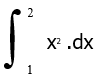Solution:

We have   I =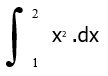We know that ∫ x2 . dx = x3 /3

Therefore, we have F(x) = x3 /3

F(2) = 23 /3 =

= 8/3 and

F(1) = 13 /3

= 1/3

so we have F(2) - F(1) = 8/3 – 1/3 = 7/3

Example: Integrate y = - x + 5 from (-2, 2)

Step 1:  set up the interval.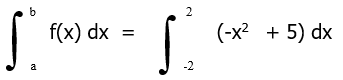Step 2:  Find the integral.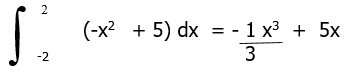Step 3:  Integrate from the given interval (-2, 2)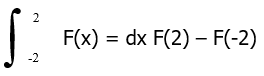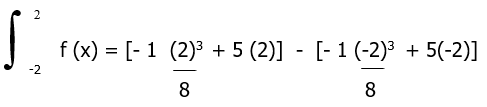=  - 8  + 10   -   8  -  10]
3                 3

=   -  [-22]
3          3

= 44/3

Therefore, the area of the curve to the x axis from -2 to 2 is 44/3 units squared.